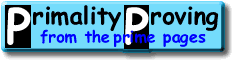Finding primes & proving primality 2.1: Finding very small primesHome > Primality Proving > Chapter Two > Small Primes

For finding all the small primes, say all those less than 10,000,000,000; one of the most efficient ways is by using the Sieve of Eratosthenes (ca 240 BC):

Make a list of all the integers less than or equal to n (greater than one) and strike out the multiples of all primes less than or equal to the square root of n, then the numbers that are left are the primes. (See also our glossary page.)

For example, to find all the odd primes less than or equal to 100 we first list the odd numbers from 3 to 100 (why even list the evens?)  The first number is 3 so it is the first odd prime--cross out all of its multiples.  Now the first number left is 5, the second odd prime--cross out all of its multiples.  Repeat with 7 and then since the first number left, 11, is larger than the square root of 100, all of the numbers left are primes.

This method is so fast that there is no reason to store a large list of primes on a computer--an efficient implementation can find them faster than a computer can read from a disk.

Bressoud has a pseudocode implementation of this algorithm [Bressoud89, p19] and Riesel a PASCAL implementation [Riesel94, p6].  It is also possible to create an even faster sieve based on quadratic forms.

To find individual small primes trial division works well.  To test n for primality (to see if it is prime) just divide by all of the primes less than the square root of n.  For example, to show is 211 is prime, we just divide by 2, 3, 5, 7, 11, and 13. (Pseudocode [Bressoud89, pp 21-22], PASCAL [Riesel94, pp 7-8].)  Sometimes the form of the number n makes this especially effective (for examples, Mersenne divisors have a special form).

Rather than divide by just the primes, it is sometimes more practical to divide by 2, 3 and 5; and then by all the numbers congruent to 1, 7, 11, 13, 17, 19, 23, and 29 modulo 30--again stopping when you reach the square root.  This type of factorization is sometimes called wheel factorization.  It requires more divisions (because some of the divisors will be composite), but does not require us to have a list of primes available.

Suppose n has twenty-five or more digits, then it is impractical to divide by the primes less than its square root.  If n has two hundred digits, then trial division is impossible--so we need much faster tests.  We discuss several such tests below.

 [ next page | previous page ] Copyright © 2023 PrimePages (Originally written by Chris Caldwell)